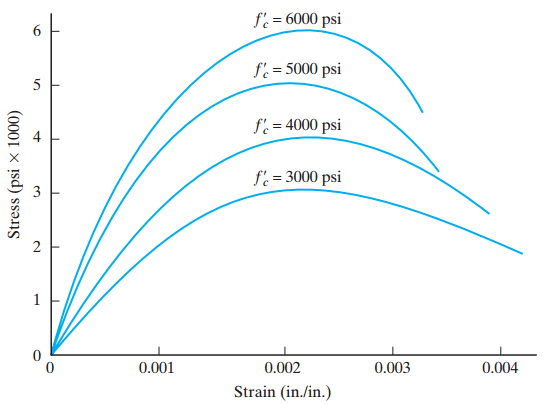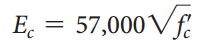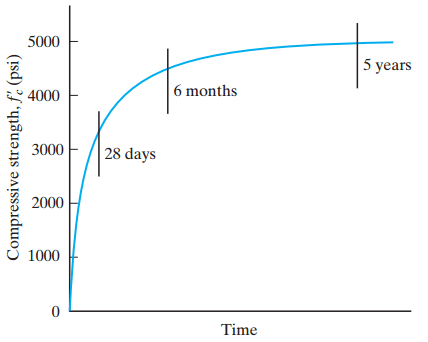## Concrete in Compression (ACI 318)## Concrete in Compression

It is generally accepted that the behavior of a reinforced concrete member under load depends on the stress–strain relationship of the materials, as well as the type of stress to which it is subjected.

With concrete used principally in compression, the compressive stress–strain curve is of primary interest.

The compressive strength of concrete is denoted fcand is assigned the units pounds per square inch (psi). For calculations, fcis frequently used with the units kips per square inch (ksi).

A test that has been standardized by ASTM C39 is used to determine the compressive strength ( fc) of concrete. The test involves compression loading to failure of a specimen cylinder of concrete. The compressive strength so determined is the highest compressive stress to which the specimen is subjected. Note in Figure 1 that fcis not the stress that exists in the specimen at failure but that which occurs at a strain of about 0.002.Figure 1 Typical stress–strain curves for concrete

Currently, 28-day concrete strengths ( fc) range  from 2500 to 9000 psi, with 3000 to 4000 psi being common for reinforced concrete structures and 5000 to 6000 psi being common for prestressed concrete members.

Concretes of much higher strengths have been achieved under laboratory conditions. The curves shown in Figure 1 represent the result of compression tests on 28-day standard cylinders for varying design mixes .

A review of the stress–strain curves for different strength concretes reveals that the maximum compressive strength is generally achieved at a unit strain of approximately 0.002 in./in. Stress then decreases, accompanied by additional strain.

Higher-strength concretes are more brittle and will fracture at a lower maximum strain than will the lower-strength concretes. The initial slope of the curve varies, unlike that of steel, and only approximates a straight line. For steel, where stresses are below the yield point and the material behaves elastically, the stress–strain plot will be a straight line. The slope of the straight line is the modulus of elasticity. For concrete, however, we observe that the straight-line portion of the plot is very short, if it exists at all. Therefore, there exists no constant value of modulus of elasticity for a given concrete because the stress–strain ratio is not constant. It may also be observed that the slope of the initial portion of the curve (if it approximates a straight line) varies with concretes of different strengths. Even if we assume a straight-line portion, the modulus of elasticity is different for concretes of different strengths. At low and moderate stresses (up to about 0.5 fc), concrete is commonly assumed to behave elastically.

The ACI Code provides the accepted empirical expression for modulus of elasticity:where
Ec = modulus of elasticity of concrete in compression (psi)
wc = unit weight of concrete (lb/ft3)
fc= compressive strength of concrete (psi)

This expression is valid for concretes having wc between 90 and 160 lb/ft3. For normal-weight concrete, the unit weight wc will vary with the mix proportions and with the character and size of the aggregates. If the unit weight is taken as 144 lb/ft3, the resulting expression for modulus of elasticity isIt should also be noted that the stress–strain curve for the same-strength concrete may be of different shapes if the condition of loading varies appreciably. With different rates of strain (loading), we will have different-shape curves. Generally, the maximum strength of a given concrete is smaller at slower rates of strain.

Concrete strength varies with time, and the specified concrete strength is usually that strength that occurs 28 days after the placing of concrete. A typical strength–time curve for normal stone concrete is shown in Figure 2. Generally, concrete attains approximately 70% of its 28-day strength in 7 days and approximately 85% to 90% in 14 days.Figure 2 Strength–time relationship for concrete.

Concrete, under load, exhibits a phenomenon termed creep. This is the property by which concrete continues to deform (or strain) over long periods of time while under constant load. Creep occurs at a decreasing rate over a period of time and may cease after several years. Generally, high-strength concretes exhibit less creep than do lower strength concretes. The magnitude of the creep deformations is proportional to the magnitude of the applied load as well as to the length of time of load application.